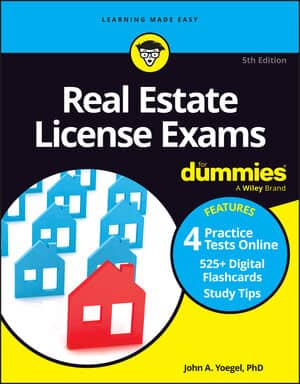##### Real Estate License Exams For DummiesA few standard problems that you may have to calculate for the Real Estate License Exam deal with mortgage interest and principal payments. Here are a few of the likely possibilities.

## Annual and monthly interest

All interest on mortgage loans is expressed as an annual interest amount, so if your mortgage interest rate is 8 percent, that’s the annual rate. But most mortgages are paid on a monthly basis, so you sometimes need to calculate how much interest you actually paid in one month based on that annual rate.

First, look at an annual interest problem.

You borrow \$100,000 at 8 percent for 30 years in an amortized mortgage loan. How much interest will you pay the first year?

Remember that in a mortgage loan, the interest rate is always quoted annually and is always based on the loan’s unpaid balance.

Loan amount x interest rate = first year’s interest

\$100,000 x 0.08 = \$8,000

The 30 years doesn’t matter. It’s extra information to confuse you.

Now here’s a monthly problem with different numbers:

You borrow \$150,000 at 8 percent interest for 30 years in an amortized loan. What is the first month’s interest on the loan?

Loan amount x interest rate = first year’s interest

\$150,000 x 0.08 = \$12,000 annual interest

You wind up with \$12,000 for the first year’s interest. To figure out the first month’s interest, all you have to do is divide the first year’s interest by 12.

First year’s interest ÷12 months = first month’s interest

\$12,000 ÷12 = \$1,000 first month’s interest

Note that this monthly interest calculation works this way only for the first month’s interest.

Test writers may go further and ask you to calculate the second month’s interest. To answer the question, you need to know what the total monthly payment is, and the test writers will tell you. In this case, the monthly payment, which includes principal and interest, is \$1,101. The question is how much is the second month’s interest payment.

\$1,101 (monthly payment) – \$1,000 (first month’s interest) = \$101 (principal payment)

\$150,000 (loan amount) – \$101 (first month’s principal payment) = \$149,899 (loan balance after first month’s payment)

\$149,899 (loan balance after first payment) x 0.08 (annual interest rate) = \$11,991.92 (interest owed for the next 12 months)

\$11,991.92 (interest owed for the next 12 months) ÷12 months = \$999.33 (interest paid for the first month of that next 12-month period, which is, in fact, the second month of the loan term of the loan)

How much is owed on the loan after the second month’s payment?

\$1,101 (monthly payment) – \$999.33 (second month’s interest) = \$101.67 (second month’s principal payment)

\$149,899 (owed after first month’s payment) – \$101.67 = \$149,797.33 (remaining loan balance after second month’s payment)

What you need to remember here is that in an amortized loan, you’re only reducing the amount you owe by the amount of principal you pay each month and not by the amount of the total payment, because payment includes interest and principal.

## Total interest

A type of interest problem that seems to confuse people is the calculation of total interest. Total interest is the amount of interest you pay during the entire life of the loan, assuming that you pay off the loan by making the payments within the required time frame. In general, banks provide these numbers to people, but you need to be familiar with this calculation for the exam.

Say you borrow \$200,000 at 7 percent for 30 years in an amortized mortgage loan. Your monthly payments are \$1,330. What is the total interest on the loan?

Most people fool around with the 7 percent for a while, but you don’t need the percentage rate of the mortgage loan to work this problem. Watch this, because you’re not going to believe how easy it is.

\$1,330 (monthly payment) x 12 months x 30 years = \$478,800 total payments during the loan’s 30-year term.

\$478,800 – \$200,000 (original loan amount) = \$278,800 interest paid during the course of the loan.

Don’t forget that every amortized loan payment contains part principal and part interest. In 30 years, you pay a total of \$478,800 in principal and interest. So if you subtract the principal, or the amount you borrowed, what you have left is interest.